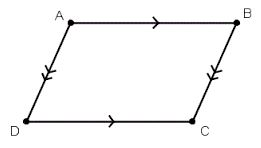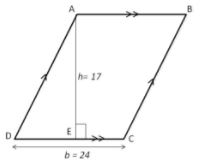# Theory - To show that the Area of Parallelogram is Product of its Base and Height, Class 9 Math Class 9 Notes | EduRev

## Class 9 : Theory - To show that the Area of Parallelogram is Product of its Base and Height, Class 9 Math Class 9 Notes | EduRev

The document Theory - To show that the Area of Parallelogram is Product of its Base and Height, Class 9 Math Class 9 Notes | EduRev is a part of the Class 9 Course Mathematics (Maths) Class 9.
All you need of Class 9 at this link: Class 9

Objective:

To show that the area of parallelogram is product of its base and height.

Theory:

A parallelogram is a simple quadrilateral with two pairs of parallel sides.Properties:

1. The opposite or facing sides of a parallelogram are of equal length.

2. The opposite angles of a parallelogram are of equal measure.

3. Opposite sides of a parallelogram are parallel (by definition) and so will never intersect.

4. The area of a parallelogram is twice the area of a triangle created by one of its diagonals.

5. Area of the parallelogram = base x height.

Example:Find the area of parallelogram ABCD with base 24 m and height 17 m.

Solution :

Area of the parallelogram = base x height = 24 X 17 =408
∴ The area of parallelogram ABCD is 408 m2

Offer running on EduRev: Apply code STAYHOME200 to get INR 200 off on our premium plan EduRev Infinity!

## Mathematics (Maths) Class 9

190 videos|233 docs|82 tests

,

,

,

,

,

,

,

,

,

,

,

,

,

,

,

,

,

,

,

,

,

,

,

,

;